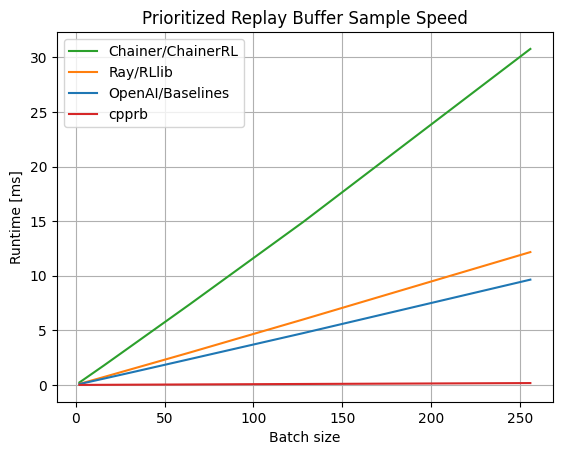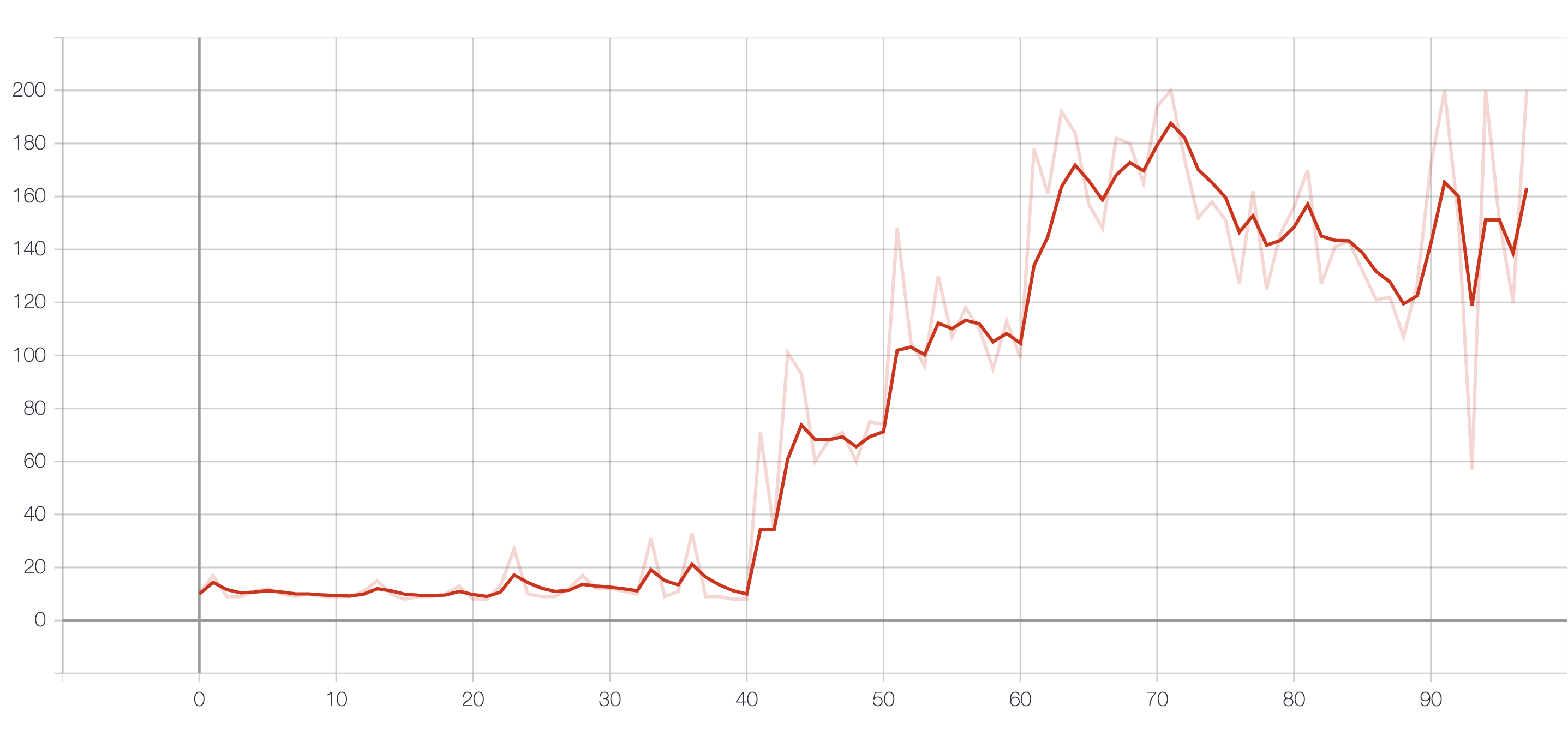# [PYTHON] [Reinforcement learning] DQN with your own library

TL;DR I implemented DQN using my own Replay Buffer library cpprb.

Recommended because it has a high degree of freedom and efficiency (I intend).

# 1. Background and background

Reinforcement learning such as Open AI / Baselines and Ray / RLlib With a set of environments, you can experiment with different algorithms with a little code.

For example, in Open AI / Baselines, the official README states that you only need to execute the following command to learn Atari's Pong with DQN.

``````python -m baselines.run --alg=deepq --env=PongNoFrameskip-v4 --num_timesteps=1e6
``````

On the other hand, it's easy to test existing algorithms, but when researchers and library developers try to create new proprietary algorithms, I think it's too big to start with.

Some of my friends who are studying reinforcement learning also use deep learning libraries such as TensorFlow, but other parts were implemented independently (it seemed).

Around the end of 2018, a friend said, "Are you interested in Cython? The Replay Buffer implemented in Python is as slow as the learning part of deep learning (depending on the situation), and I want to speed up with Cython." It was cpprb that I was invited to implement (memory).

(The friend used cpprb and TensorFlow 2.x to publish a reinforcement learning library called tf2rl, which is also highly recommended!)

# 2. Features

Against this background, cpprb has begun to be implemented, so we are developing it with a focus on high degree of freedom and efficiency.

## 2.1 High degree of freedom

You can freely decide the variable name, size, and type to be saved in the buffer by specifying them in the `dict` format.

For example, in the extreme case, you can save `next_next_obs`,` previous_act`, `secondary_reward`.

``````import numpy as np
from cpprb import ReplayBuffer

buffer_size = 1024

#shape and dtype can be specified for each variable. The default is{"shape":1,"dtype": np.float32}
rb = ReplayBuffer(buffer_size,
{"obs": {"shape": (3,3)},
"act": {"shape": 3, "dtype": np.int},
"rew": {},
"done": {},
"next_obs": {"shape": (3,3)},
"next_next_obs": {"shape": (3,3)},
"previous_act": {"shape": 3, "dtype": np.int},
"secondary_reward": {}})

# Key-Specify in Value format (if the variable specified at initialization is insufficient`KeyError`）
act=np.ones(3,dtype=np.int),
rew=0.5,
done=0,
next_obs=np.zeros(shape=(3,3)),
next_next_obs=np.ones(shape=(3,3)),
previous_act=np.ones(3,dtype=np.int),
secondary_reward=0.3)
``````

## 2.2 Efficiency

It is quite fast because the Segment Tree, which is the cause of the slowness of Prioritized Experience Replay, is implemented in C ++ via Cython.As far as the benchmark is seen, it is overwhelmingly fast. (As of April 2020. For the latest version, go to Project Site)

Note: For reinforcement learning as a whole, not only the speed of the Segment Tree but also measures such as successfully parallelizing the search are important.

# 3. Installation

(See also the latest install method as the information may be out of date)

## 3.1 Binary installation

It's published on PyPI, so you can install it using pip (or a similar tool).

Since the wheel format binary is distributed for Windows / Linux, in many cases you can install it with the following command without thinking about anything. (Note: It is recommended to use virtual environment such as `venv` and docker.)

``````pip install cpprb
``````

Note: macOS is part of the standard development toolchain clang, but instead of the C ++ 17 feature `std :: shared_ptr` array type Since the specialization of is not implemented, it cannot be compiled and the binary cannot be distributed.

## 3.2 Install from source

You need to build from the source code yourself. You need the following to build:

• GCC >= 7.2(?)

You need to run the build with `g ++` in the environment variables `CC` and` CXX`.

# 3. DQN implementation

I wrote a DQN that works on Google Colab

First, install the required libraries

``````!apt update > /dev/null 2>&1
!apt install -y xvfb x11-utils python-opengl > /dev/null
!pip install gym cpprb["all"] tensorflow > /dev/null
``````
``````import os
import datetime
import io
import base64

import numpy as np

from google.colab import files, drive

import gym

import tensorflow as tf
from tensorflow.keras.models import Sequential,clone_model
from tensorflow.keras.layers import InputLayer,Dense
from tensorflow.keras.optimizers import Adam
from tensorflow.keras.callbacks import EarlyStopping,TensorBoard
from tensorflow.summary import create_file_writer

from scipy.special import softmax
from tqdm import tqdm_notebook as tqdm

from cpprb import create_buffer, ReplayBuffer,PrioritizedReplayBuffer
import cpprb.gym
``````
``````JST = datetime.timezone(datetime.timedelta(hours=+9), 'JST')
a = cpprb.gym.NotebookAnimation()
%tensorboard --logdir logs
``````
``````#DQN test:Modeling

gamma = 0.99
batch_size = 1024

N_iteration = 101
N_show = 10

per_train = 100

prioritized = True

egreedy = True

loss = "huber_loss"
# loss = "mean_squared_error"

dir_name = datetime.datetime.now(JST).strftime("%Y%m%d-%H%M%S")

logdir = os.path.join("logs", dir_name)
writer = create_file_writer(logdir + "/metrics")
writer.set_as_default()

env = gym.make('CartPole-v0')
env = gym.wrappers.Monitor(env,logdir + "/video/", force=True,video_callable=(lambda ep: ep % 50 == 0))

observation = env.reset()

model = Sequential([InputLayer(input_shape=(observation.shape)), # 4 for CartPole
Dense(64,activation='relu'),
Dense(64,activation='relu'),
Dense(env.action_space.n)]) # 2 for CartPole

target_model = clone_model(model)

tensorboard_callback = TensorBoard(logdir, histogram_freq=1)

model.compile(loss =  loss,
optimizer = optimizer,
metrics=['accuracy'])

a.clear()

rb = create_buffer(1e6,
{"obs":{"shape": observation.shape},
"act":{"shape": 1,"dtype": np.ubyte},
"rew": {},
"next_obs": {"shape": observation.shape},
"done": {}},
prioritized = prioritized)

action_index = np.arange(env.action_space.n).reshape(1,-1)

#Random initial search
for n_episode in range (1000):
observation = env.reset()
sum_reward = 0
for t in range(500):
action = env.action_space.sample() #Random selection of actions
next_observation, reward, done, info = env.step(action)
observation = next_observation
if done:
break

for n_episode in tqdm(range (N_iteration)):
observation = env.reset()
for t in range(500):
if n_episode % (N_iteration // N_show)== 0:

actions = softmax(np.ravel(model.predict(observation.reshape(1,-1),batch_size=1)))
actions = actions / actions.sum()

if egreedy:
if np.random.rand() < 0.9:
action = np.argmax(actions)
else:
action = env.action_space.sample()
else:
action = np.random.choice(actions.shape,p=actions)

next_observation, reward, done, info = env.step(action)
sum_reward += reward
act=action,
rew=reward,
next_obs=next_observation,
done=done)
observation = next_observation

sample = rb.sample(batch_size)
Q_pred = model.predict(sample["obs"])
Q_true = target_model.predict(sample['next_obs']).max(axis=1,keepdims=True)*gamma*(1.0 - sample["done"]) + sample['rew']
target = tf.where(tf.one_hot(tf.cast(tf.reshape(sample["act"],[-1]),dtype=tf.int32),env.action_space.n,True,False),
Q_pred)

if prioritized:
TD = np.square(target - Q_pred).sum(axis=1)
rb.update_priorities(sample["indexes"],TD)

model.fit(x=sample['obs'],
y=target,
batch_size=batch_size,
verbose = 0)

if done:
break

if n_episode % 10 == 0:
target_model.set_weights(model.get_weights())

tf.summary.scalar("reward",data=sum_reward,step=n_episode)

rb.clear()
a.display()
``````

# 4. Result

The result of reward.# 6. Summary

I implemented DQN using my own library cpprb that provides a Replay Buffer for reinforcement learning.

cpprb is developed with a high degree of freedom and efficiency.

If you're interested, give it a try and submit an issue or merge request. (English is preferable, but Japanese is also OK)